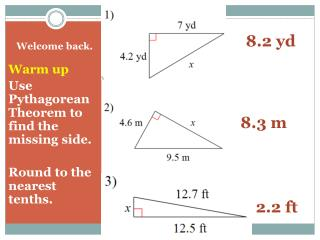Download PresentationWelcome back.Welcome back. - PowerPoint PPT Presentation

Download PresentationWelcome back.
An Image/Link below is provided (as is) to download presentation

Download Policy: Content on the Website is provided to you AS IS for your information and personal use and may not be sold / licensed / shared on other websites without getting consent from its author. While downloading, if for some reason you are not able to download a presentation, the publisher may have deleted the file from their server.

- - - - - - - - - - - - - - - - - - - - - - - - - - - E N D - - - - - - - - - - - - - - - - - - - - - - - - - - -
Presentation Transcript

1. Warm up Use Pythagorean Theorem to find the missing side. Round to the nearest tenths. 8.2 yd Welcome back. 8.3 m 2.2 ft

2. TANGENTS A line is tangent to the circle only if it is perpendicular to the radius drawn to the point of tangency

3. Is AD tangent or not tangent? No, point D is not tangent to the circle.

4. Is AB tangent or not tangent?Use Pythagorean Theorem to find out. Yes 682 + 512 = 852

5. Is AB tangent or not tangent?Use Pythagorean Theorem to find out. No 82 + 162 192

6. Solve for x. x2 + 962 = 1002 x = 28 BC is a radius of Circle C AB is tangent to Circle C

7. Solve for x. x2 + 482 = 802 x = 64 BC is a radius of Circle C AB is tangent to Circle C

8. Solve for x. x2 + 322 = (x + 16)2 32x = 768 x = 24 BC is a radius of Circle C AB is tangent to Circle C

9. Solve for x. x2 + 562 = (x + 32)2 64x = 2112 x = 33 BC is a radius of Circle C AB is tangent to Circle C

10. Solve for x. x2 + 992 = (x + 81)2 162x = 3240 x = 20 BC is a radius of Circle C AB is tangent to Circle C

11. P. 207

12. Solve for x.  7.1 x 6

13. EF EG

14. Solve for x. Round to the nearest tenth.

15. Party Hat Problems(Tangent/Tangent)

16. S Party hat problems! If two segments from the same exterior point are tangent to a circle, then they are congruent. R T

17. Solve for x

18. Solve for x

19. Solve for x C P

20. Find NP N T S P R Q